# Model Verification

This page builds upon the System Modeling section described previously. In this section, we estimate the model parameters based on our physical system. This will not only improve the accuracy of our simulated model, but will also help us identify accurate parameters for our control systems. The more accurate the initial control parameters, the less time needed to spend tuning the values.

###### Physical Model Parameters

These parameters are based off of the 3DR ArduCopter Quad-C Frame DIY kit. The moments of inertia can be calculated several ways. In the equations below, the stack-up of the frame is modeled a hollow sphere. The stack-up is the center of the quadrotor which holds the APM 2.6+ autopilot system, 3DR uBlox GPS and compass4000mAh 3S 30C LiPo battery, APM Power Module, and Power Distribution Board. We also add the weight of four 20 Amp ESCs, a Spektrum AR8000 Radio Receiver, and the 3DR 433MHz telemetry radio. We model the 880Kv motors as cylinders and use Huygens–Steiner to move the reference axis of the cylinders towards the sphere. These equations as well as the appropriate values are computed below. Note that the quadrotor can be modeled using other geometric shapes defined in this moment of inertia table.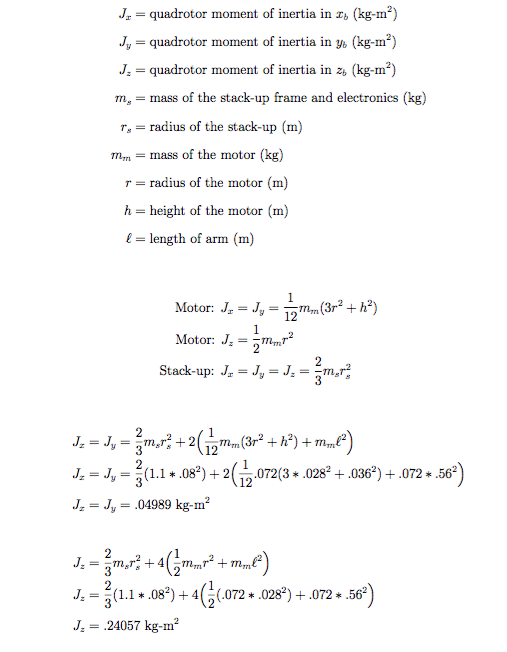Propeller Moment of Inertia

To calculate the moment of inertia of the propellers, it is possible to use the work of Tomas Jiinec’s Master’s thesis. Specifically, section 4.1.1 calculates the motor of inertia for an APC 10×4.7 propeller which is nearly identical to the APC 11×4.7 propellers used for this project. He measures the weight and density of the propeller, divides the propeller into sections, and estimates the total moment of inertia as the sum of the sections using the parallel axis theorem. He estimates the moment of inertia to be 0.04439 kg-m^2.

###### Sensor Characterization

The sensors below came standard with the APM 2.6+ autopilot board. In order to improve our simulation and therefore, controller design, we need to accurately simulate the performance of the sensors. These parameters will be used to add error to the simulated sensor measurements. We can then use a filter to reduce the impact of sensor noise while fusing the various sensor measurements together to calculate more accurate position and attitude information. This page has relevant parameters for other types of sensors not discussed below. The manufacturer’s datasheet information is detailed below followed by a discussion describing how to manually estimating the sensor parameters.

3DR uBlox LEA-6 GPS with HMC5883L Digital Compass Kit (GPS Datasheet, Compass Datasheet)

• GPS: 5 Hz update rate, 2.5m CEP
• Compass: 160 Hz Maximum Output Rate, 1° to 2° heading accuracy, 4.3 mG/digit sensitivity

MPU-6000 6-Axis Gyro+Accel (Datasheet)

• Gyroscope: 8kHz maximum output rate, 16.4 LSB/°/sec sensitivity, 0.05 °/sec noise
• Accelerometer: 1kHz maximum output rate,  2,048 LSB/g sensitivity, 400 ug/√Hz noise density
MS5611-01BA03 Barometric Pressure Sensor (Datasheet)
• 2kHz maximum output rate, 10cm altitude resolution, +/- 0.5 Hpa relative accuracy

Optional Sensors:

MB1240 XL-MaxSonar®-EZ4TM High Performance Ultrasonic Range Finder (Datasheet)

• 10Hz sample rate, 1cm resolution

Rate Gyroscope Modeling:

A MEMS rate gyroscope contains a small vibrating lever. To detect rotation, the change of frequency of the lever due to the Coriolis effect is measured when the lever is rotated. If the gyros are aligned with the x, y, and z axis of the quadrotor then the gyros measure the angular body rates p, r, and r.

The gyro can be modeled as a combination of the true measurement, a bias, and noise. The true angular rate is measured in radians per second. The noise is characterized as zero mean white Gaussian noise. The bias is dependent on temperature and can be estimated for each flight. These elements produce the following equations to model the gyroscope. The same model is used for each axis.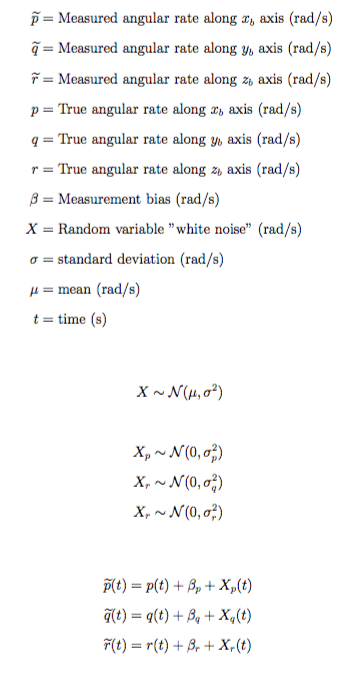In order to estimate the bias and the standard deviation of the white noise, the IMU was run at 100Hz for 10 minutes. The results were recorded in a .csv file using this Arduino .pde file to record the sensor data. Next, a Matlab script was run to compute the noise characteristics and to plot the recorded gyroscope measurements and the modeled gyroscope measurements. Both of these outputs are shown below.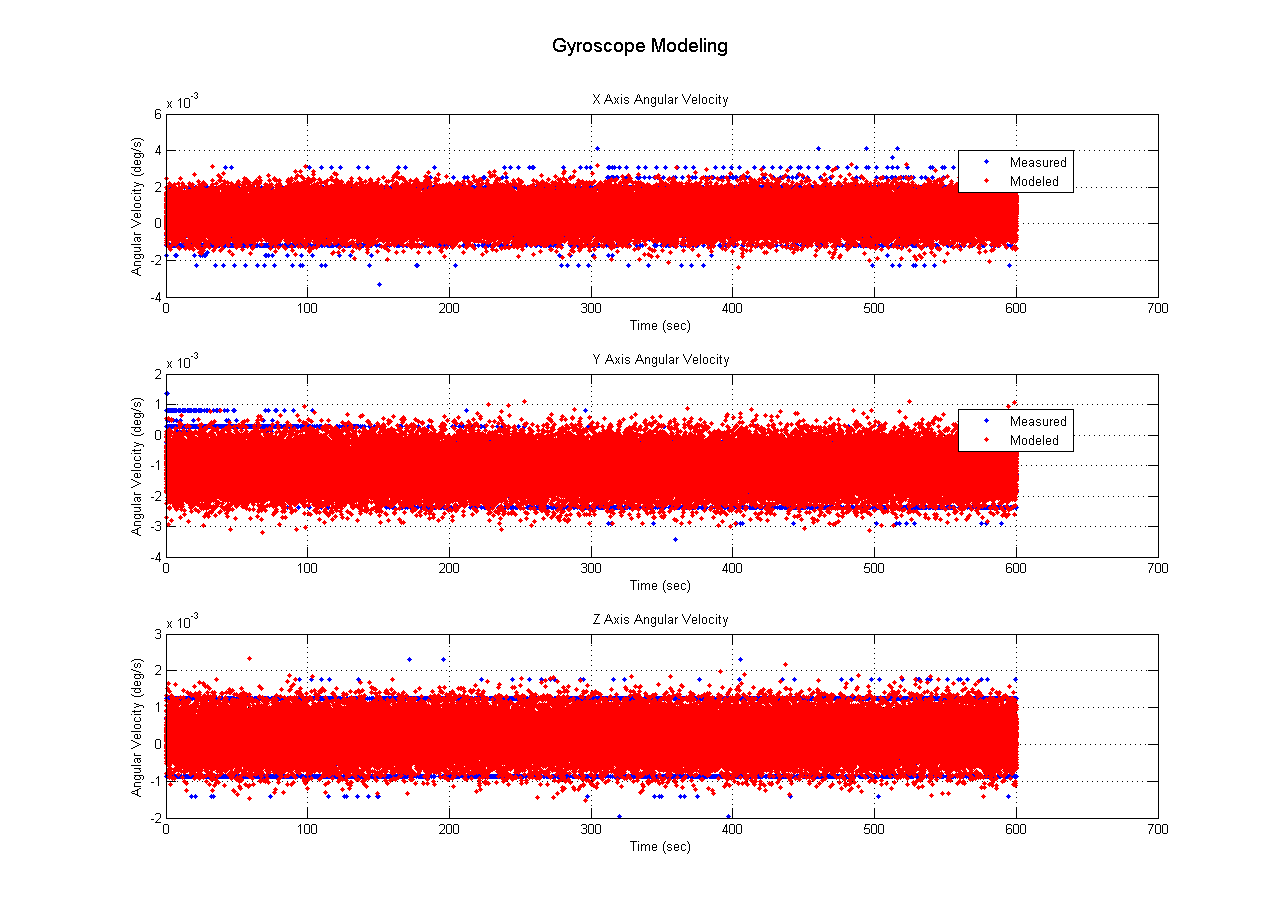Accelerometer Modeling:

A MEMS accelerometer contains torsion levers attached to a small plate. The plate rotates under acceleration which changes the capacitance between the plate and the surrounding walls. If the accelerometer is aligned with the x, y, and z axis of the quadrotor then the accelerometer output is the linear acceleration in the body frame.

The accelerometer can be modeled as a combination of the true measurement, a bias, and noise. The true linear acceleration is measured in meters per second squared. The noise is characterized as zero mean white Gaussian noise. The bias is dependent on temperature and can be estimated for each flight. These elements produce the following equations to model the gyroscope. The same model is used for each axis.The accelerometer noise characteristics were estimated in the same manner as the gyroscope. Measurements were recorded at 100 Hz using the Arduino and post-processed in Matlab. The noise characteristics and a comparison of the measured and modeled acceleration values is shown below.

For both the gyroscope and the accelerometer, simplifications were made to develop the model. First, each sensor was stationary as the data was collected. In reality, we expect these sensors to be in constant motion. Also, the sensors were measured at a constant temperature. In reality, changes in altitude will result in temperature changes which will impact the sensor biases. Both of these factors are not accounted for in this simple model.

###### Motor System Parameters

Brushless DC motors such as the four 880Kv motors used on this quadrotor are popular with quadrotors because they are small motors with high torque. These motors are considered outrunners since the rotating part is on the outside and not the inside. This allows the motors to generate higher torque. This high torque to weight ratio results in a high efficient factor which requires less battery weight and more flight time between charges. The high torques allow the motors to change speed faster, which ultimately controls the motion of the quadrotor. The high torque also eliminates the need for a gearbox which further reduces weight.

The basic physical principles used to describe a conventional permanent magnet DC motor can be applied to the brushless DC motor. The simplified electrical equation (Kirchoff’s Law) and the dynamic equation for the motor coupled to a load (Newton’s Second Law) is defined below in the time domain. Additionally, the back emf is proportional to the angular velocity of the motor. Faraday’s law can be used to describe the voltage created by the changing magnetic field in a coil. Lastly, Lorentz’s law which describes the force upon a coil in a magnetic field results in the produced torque being proportional to the motor current. These equations are shown below in the time domain.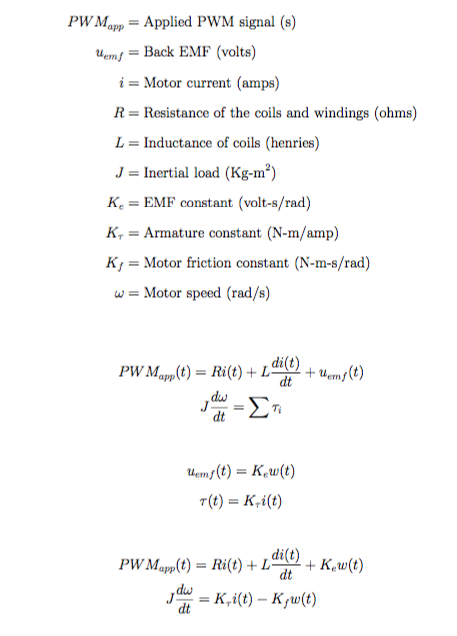Motor Dynamics Identification

In order to model the system dynamics of the motor, we need to calculate the transfer function that describes the motor dynamics. The transfer function’s output is the propeller speed and the input is the motor voltage. The transfer function will allow us to calculate the time constant of the motor and the DC gain. In the equations below, the equations are converted from the time domain to the frequency domain using the Laplace transform.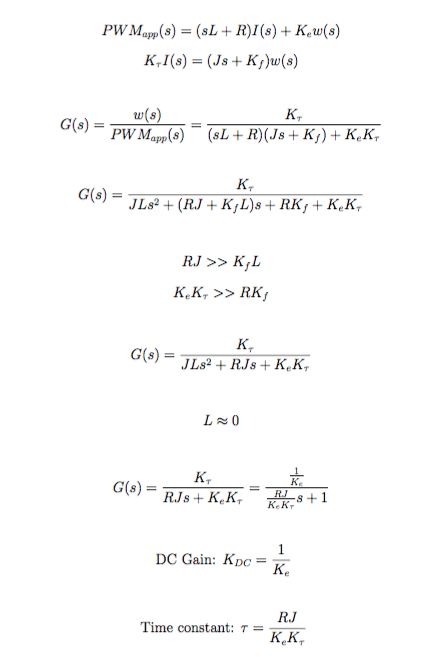Several assumptions are made to reduce the transfer function to approximate a first order system. The force due to friction in a brushless DC motor is primarily due to bearing drag and is thus very small. Also, since the motor is small, we can assume the inductance is low and neglect this term as well. This results in the transfer function above. Additionally, the equations for the time constant and DC gain are calculated.

Instead of attempting to measure the individual variables, an experiment was developed to measure the motor response and estimate the motor parameters. The transfer function’s input is the motor voltage and the output is the speed of the motor shaft. We can control the motor input using the ESCs and measure the propeller speed using a photointerrupter.

An Arduino Uno was used to control the motor input signal and record the propeller speed. This is a popular micro-controller based on the ATmega328Pchipset. It includes multiple digital and analog input and output pins as well as a USB connection. The idea is to send the ESC varying inputs using a PWM out pin which are then translated to voltages to the motor. The photointerrupter uses an LED and an optical receiver to create a simple circuit. When an object passes between the LED and the receiver, the electric circuit is broken. We can use this sensor to detect the propeller each time it breaks the circuit, record the timestamp, and calculate the propeller speed. This data can then be analyzed using a MATLAB script to estimate the DC gain and time constant and verify the motor model against the recorded measurements.

This blog post describes how to set up the electronics circuit. Of note is the size of the pull down resistor. Since we will be reading the photointerrupter input on a digital input pin, this resistor helps pull the circuit voltage down to ground when the circuit is open, creating a clearer distinction between an open and closed circuit, recorded as a 0 or 1. The fritzing diagram below shows the complete electronics set-up using parts developed from here and here.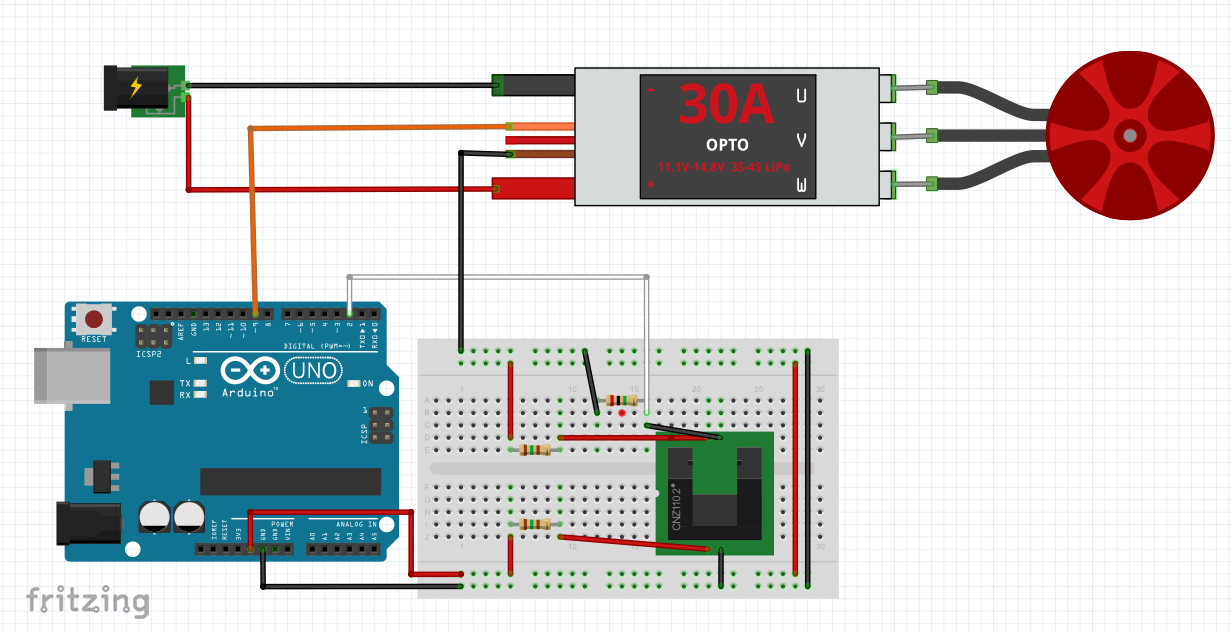While we used some assumptions to simplify the motor equations, the actual system is nonlinear due to aerodynamic drag forces on the propeller. Therefore, the model above is known as a Linear Time Invariant system. Since the equations are linearized, the model will only be accurate around the chosen setpoint. The hovering setpoint is chosen for the linearization point. Therefore, when we send motor commands in our experiment, we will keep the values in the vicinity of the hovering setpoint input value.

From previously calibrating the motors, we know that the minimum throttle value is around 1000 microseconds and the maximum throttle value is around 2000 microseconds. By flying the quadrotor manually near hover, our setpoint input is around 1425 microseconds. An Arduino script then sends motor commands around 1425 for 5 seconds each. An interrupt is used to measure the time when the propeller passes through the photointerrupter and breaks the circuit. The output of this experiment is a .csv file with columns containing the timestamp, PWM input, and propeller speed.

We then utilize a Matlab script to input the .csv file and parse the data. A transfer function is developed in the form shown above with the DC gain and time constant as variables. The input to the transfer function is the set of PWM commands sent to the actual motor in the experiment. The output of the transfer function is the corresponding simulated RPM of the propeller. These values are plotted against the experimental values in order to determine the unknown system parameters. Using a time constant of 0.15 seconds and a DC gain of 6.5, the simulated RPM values match closely to the experimental values, validating the model.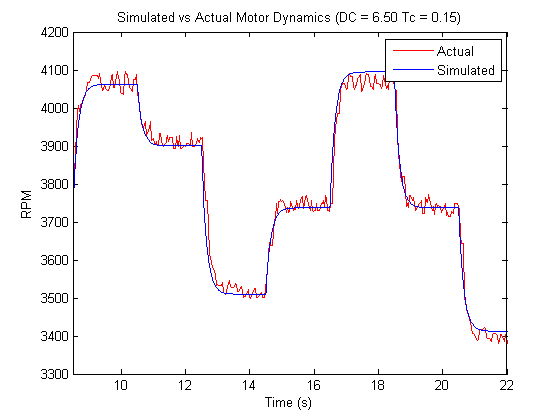In order to implement the motor model using actual PWM input data, the transfer function had to be converted from a continuous time to a discrete time model. This was done using the Matlab c2d command. A zero order hold approximation was used as well as a PWM sample time of 30 Hz calculated from the recorded flight data. The calculations to convert the model from the frequency domain to the time domain, and to calculate the final difference equation are show below. The z transform is used to calculate the difference equation. The z transform is used in digital control systems similar to the way the Laplace transform was used earlier in the continuous-time control systems. The difference equation is used to estimate the motor speed in real time given the current PWM values. Since the motor speeds are the input to the quadrotor equations of motion, this equation allows us to estimate the motor speed from our previously recorded flights to refine our quadrotor model.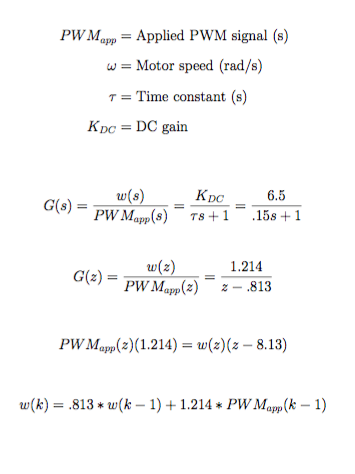Thrust Coefficient

The thrust coefficient can be estimated using the motor parameters defined above. Recall that the thrust coefficient is a proportional constant that relates the thrust of each motor to the square of the angular rate of the propeller. This is easiest to calculate at the hover point since we know that the thrust of each motor is equal to a quarter of the mass of the quadrotor and we can estimate the propeller speed from the data previously collected. The calculation of the thrust coefficient is detailed below.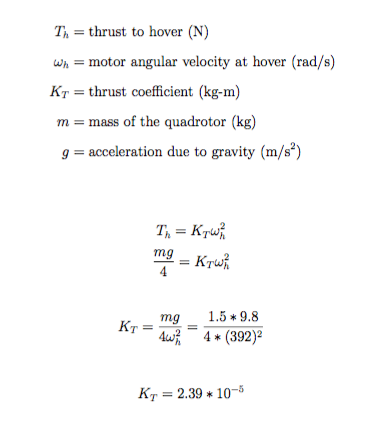Maximum motor speed:

To improve the accuracy of the simulation, we can introduce limits on the motor speed. This will prevent the control system from calculating control signals that are not realistic on the actual quadrotor system. Placing saturation limits on the motor speed also assists in tuning the control gains and prevents the utilization of control gains that are unrealistically high. The PWM commands create a duty cycle which can be correlated to the amount of voltage being sent to the motor. From our previous experiments, we know that the quadrotor hovers near a PWM command of 1425 which corresponds to a voltage of around 5.35 volts. For this given PWM signal, we estimate the propeller to be spinning at around 3745 revolutions per minute. From the motor data sheet, the unloaded motor velocity constant (Kv) is 880 RPM/V. Our loaded Kv is 700. Looking at the motor data sheet, each motor produces 1380g of thrust pulling 20A and 210W at full power which equals 10.5 volts. Therefore, we can expect the propeller to spin no faster than 7,350 RPM.

###### Parameter Estimation Through Simulation

This section will utilize known ground truth measurements to estimate unknown system parameters and validate the systems parameters estimated in the previous sections. Our model is complex, with many unknown coefficients having an impact on the quadrotor’s performance. In order to estimate these coefficients and validate our model, we can compare the output of our simulated model with known ground truth measurements. To get the ground truth measurements, a Vicon motion capture system was used. This system records the position and attitude of the quadrotor to a very high degree of accuracy and at a high sampling rate. Using this system, the quadrotor was flown in the lab with the Vicon recording the attitude and position information and the quadrotor autopilot recording the RC inputs, IMU outputs, and the ESC motor commands. The values recorded by the autopilot were used as inputs to the simulated model to validate our equations of motion of the quadrotor system. All of this data was processed using a Matlab script.

The first portion of the system identification attempted to determine the total quadrotor system delay from RC input to system attitude. Basically, how long does it take the quadrotor to receive a command and move to that desired position? To do this, we compare the RC inputs (desired roll, pitch, yaw velocity, and thrust) to the roll, pitch, yaw velocity and vertical acceleration measured by Vicon. We can then alter the timestamps of the RC input to determine at which offset the RC inputs best align with the Vicon measurements. This offset is the estimated delay in the quadrotor system. A plot of this comparison is shown below.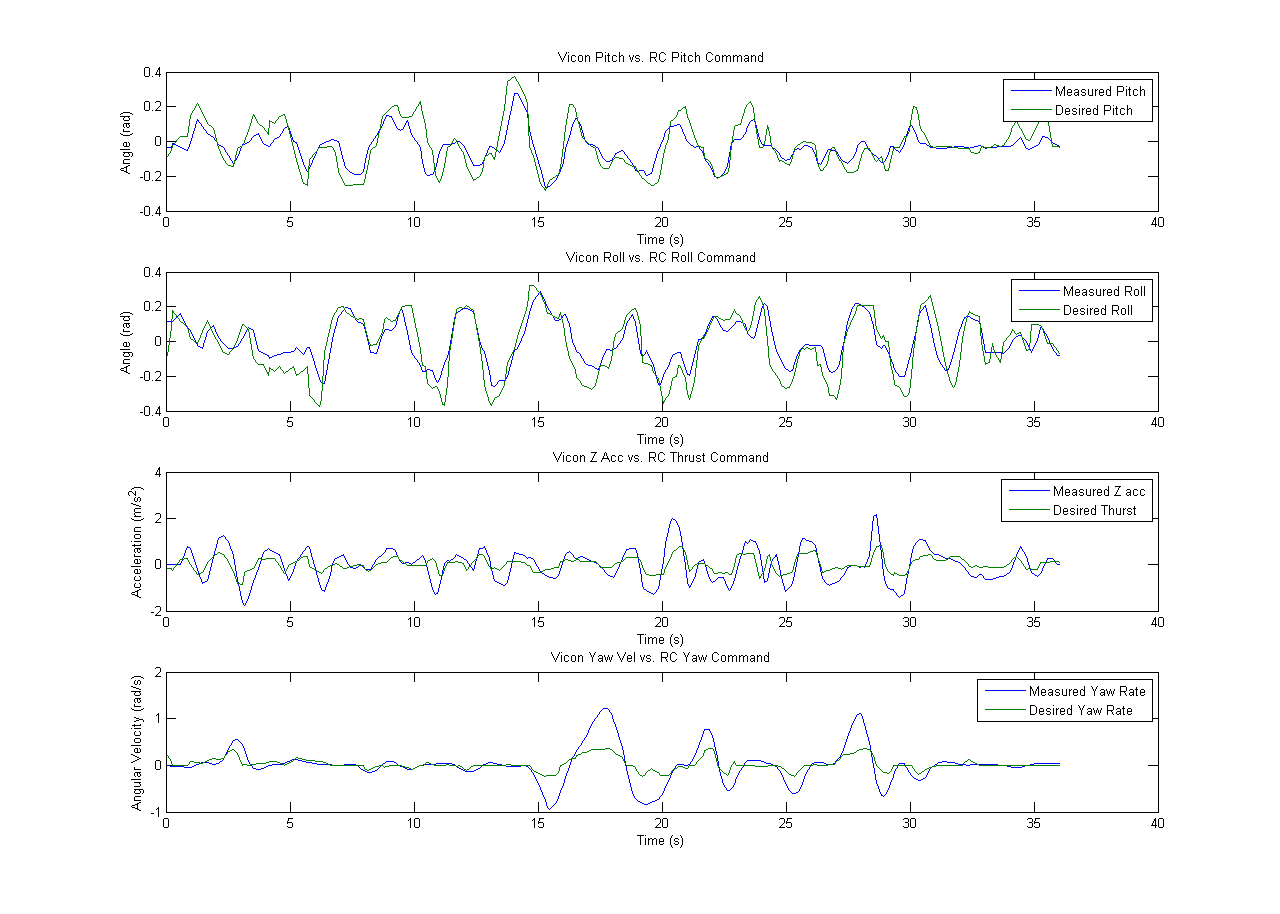Next, we investigated the translation acceleration equations of motion. We used the equations of motion developed in the System Modeling section and compared the outputs to the accelerations measured by Vicon. A simple regression was run on the output of the model to calculate the best fit coefficients. The IMU angles and estimated motor speeds were inputs into the model as well as the estimated coefficients. From the plot below, we can see that the model matches up fairly well with the measured accelerations.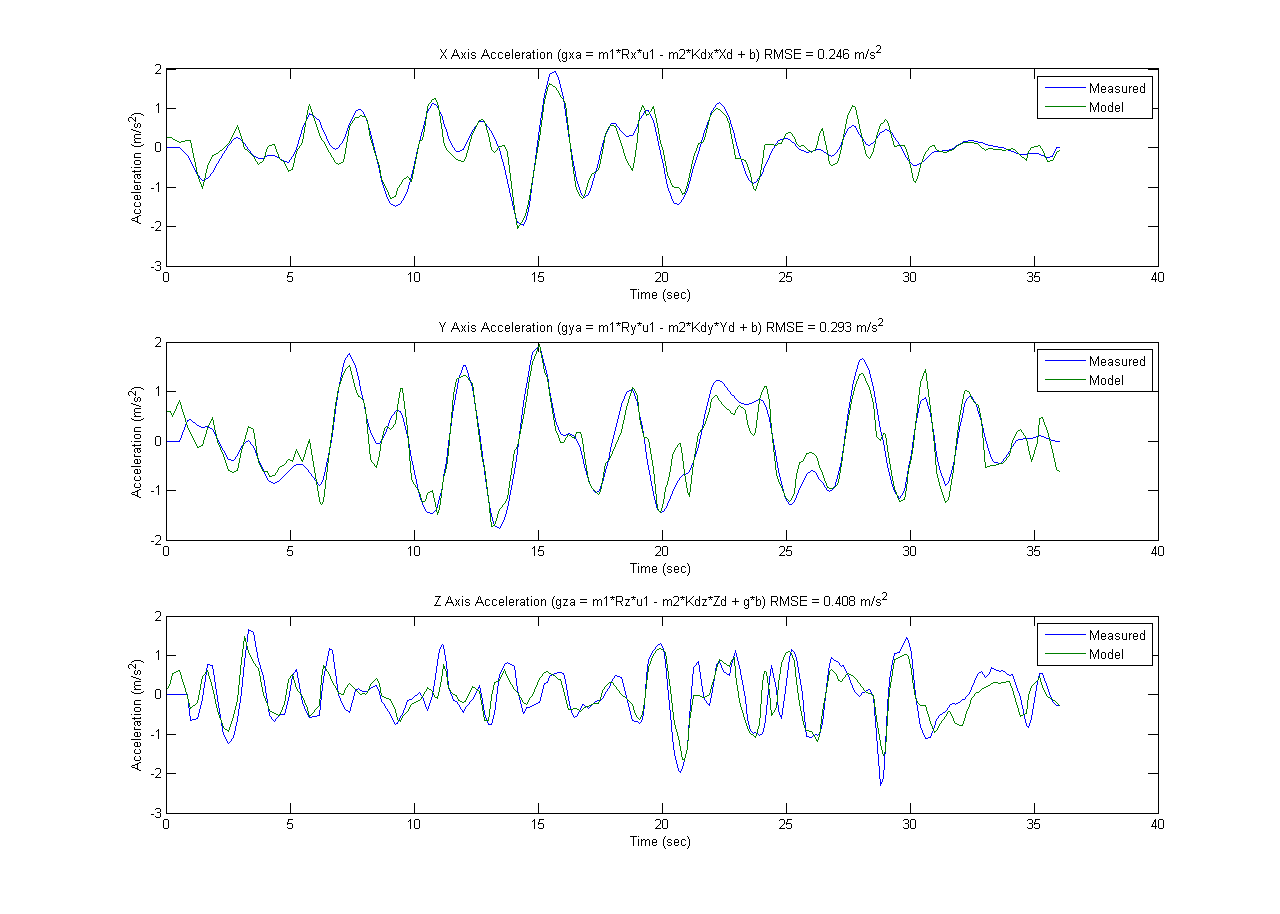Lastly, we performed similar calculations on the rotational acceleration equations of motion. Again, the IMU measurements and the estimated propeller speeds were input into the equations developed in the System Modeling section. A regression was run on the model output, comparing the values to the Vicon measurements to determine the coefficient. Unfortunately, since most of the coefficients in the rotational dynamics are coupled together, it was not possible to estimate individual unknown parameters. However, the results are reasonable and the general coefficients can be used from the model for controller design purposes as desired. A plot of the modeled and measured angular accelerations is shown below.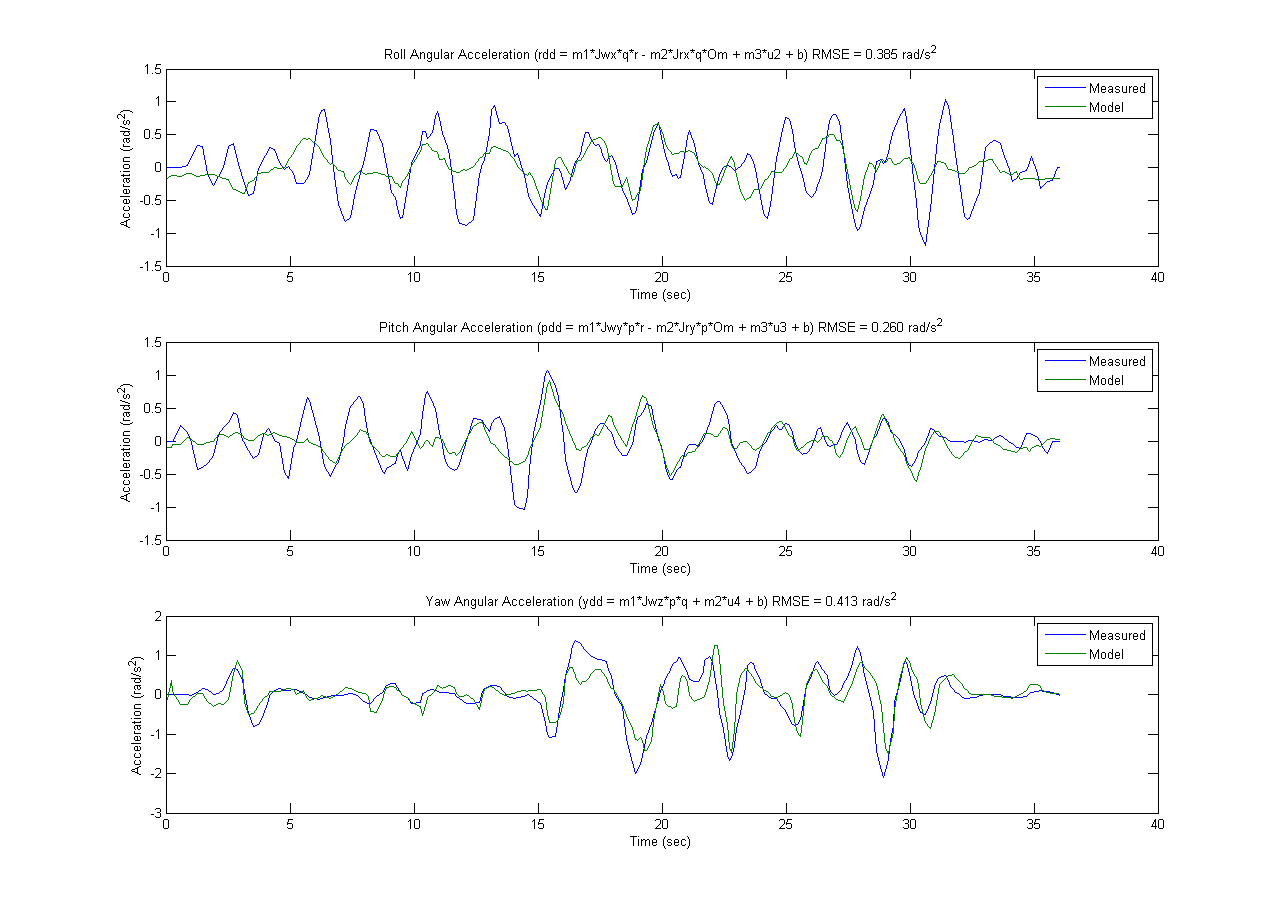While this model doesn’t align perfectly, it does capture the non-linear dynamics of the quadrotor system. In order to utilize this model for the controller simulation, we need to record the refined system parameters. The final measured or learned parameters are depicted below.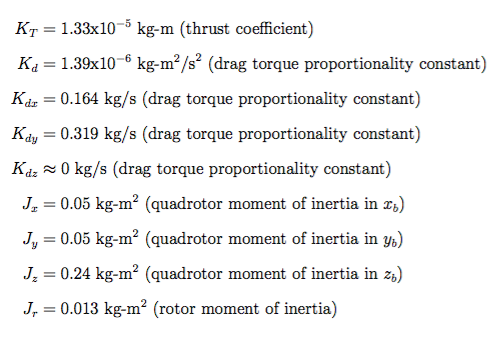Resources:

Johan Wiberg, “Controlling a Brushless DC Motor in a Shift-by-Wire System”

Petko Petkov, Tsonyo Slavov, “Stochastic Modeling of MEMS Inertial Sensors”

Tomáš Jiřinec, “Stabilization and Control of an Unmanned Quadcop

## 2 thoughts on “Model Verification”

1.khaled says:

very good woke man I am so happy about you

2.vasanthakumar v says:

Sir, Thank you very much for sharing your work ,In parameter calculation Drag coefficient calculation was not discussed can you please post the same or mail to me if possible [email protected] thank you sir.